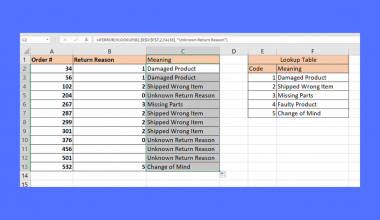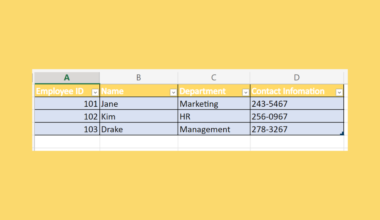# How to Sum Filtered Rows in Excel

This guide will discuss how to sum filtered rows in Excel using three simple and easy ways.

Excel is an excellent tool to use for different purposes and situations. Moreover, we can easily perform complex and long calculations using its built-in functions and tools. And we can manipulate and filter our data set.

When we filter rows or columns in our data set, we do not have to worry about performing calculations on them. In Excel, we can still easily perform calculations on filtered rows or columns.

In this guide, we will focus on learning how to sum filtered rows in Excel. When we filter rows in our data set only to show specific values or hide unwanted values, we may think that we cannot sum the values anymore. However, there are easy and simple methods to sum values in filtered rows.

Firstly, we can use the `SUBTOTAL` function to get the sum of the filtered rows. Secondly, we can also utilize the `AGGREGATE` function to sum filtered rows in Excel. Lastly, we can turn our data set into a table and obtain the sum of the filtered rows.

Let’s take a sample scenario wherein we need to sum filtered rows in Excel.

Suppose you have created a data set for the products sold in your store. So you have two columns containing the product name and amount sold. Let’s say you only need to sum the total amount sold for a specific product.

To do this, you would need to filter the data set to isolate the product you want. Then, you can use the `SUBTOTAL` function to get the sum of the filtered product.

Before we move on and dive into the methods of how to sum filtered rows in Excel, let’s first learn how to write the functions we need to use.

## The Anatomy of the SUBTOTAL Function

The syntax or the way we write the `SUBTOTAL` function is as follows:

`=SUBTOTAL(function_num, ref1,...)`

Let’s take apart this formula and understand what each term means:

• = the equal sign is how we begin any function in Excel.
• SUBTOTAL() refers to our `SUBTOTAL` function. And this function is used to return a subtotal in a list or database.
• function_num is a required argument. So this refers to the number 1 to 11 that will specify the summary function for the subtotal.
• ref1 is another required argument. And this refers to 1 to 254 ranges or references for which we want to get the subtotal.

## The Anatomy of the AGGREGATE Function

The syntax or the way we write the `AGGREGATE` function is as follows:

`=AGGREGATE(function_num, options, array, [k],...)`

Let’s take apart this formula and understand what each term means:

• = the equal sign is how we start any function in Excel.
• AGGREGATE() is our `AGGREGATE` function. And this function is used to return an aggregate in a list or database.
• function_num is a required argument. So this refers to the number 1 to 19 that will specify the summary function for the aggregate.
• options is another required argument. And this refers to the numbers 0 to 7 that will specify the values to ignore for the aggregate.
• array is also a required argument. So this refers to the array or range of numerical data on which to calculate the aggregate.
• k is an optional argument. And this value will indicate the position in the array. For instance, it can be k-th largest, k-th smallest, k-th percentile, k-th quartile.

Great! Now we can dive into the process of how to sum filtered rows in Excel using three simple and easy ways.

## How to Sum Filtered Rows in Excel Using SUBTOTAL

Firstly, we can use the `SUBTOTAL` function to sum filtered rows in Excel. So the `SUBTOTAL` function will return the `SUBTOTAL` of the selected range or array. In this case, we will first determine the `SUBTOTAL` of our entire data set.

Then, we will add a filter to our data set. Afterward, we can filter the data set to only show the data we want. Furthermore, the `SUBTOTAL` value will automatically update to the filtered values.

To apply this method, we can simply follow the steps below.

1. Firstly, we will create an area for the sum value and input the `SUBTOTAL` formula there. To do this, we will type the formula “=SUBTOTAL(9, C2:C8)”. Lastly, we will press the Enter key to return the result.2. Secondly, we will select the entire data set and go to the Data tab. Next, we will click Filter found in the Sort&Filter section.3. Thirdly, we can filter out the product by clicking the dropdown arrow beside Product Name. Afterward, we can simply check the product we want to filter and click Apply.4. And tada! We have successfully summed filtered rows in Excel.## How to Sum Filtered Rows in Excel Using AGGREGATE

Secondly, we can utilize the `AGGREGATE` function to sum filtered rows in Excel. So the `AGGREGATE` function will return the aggregate of the selected range. In this case, we will input the function to ignore the hidden rows meaning the rows we filtered out will not be included in the calculation.

To apply this method, we can simply follow the steps below.

1. Firstly, we will create a cell to input the result. Then, we will type in the formula “=AGGREGATE(9, 5, B2:C8)”. Lastly, we will press the Enter key to return the result.2. Secondly, we can select the entire data set and go to the Data tab. Next, we will click Filter.3. Thirdly, we will click the dropdown arrow beside the header. Then, we will check the specific product we want to filter out. Finally, we will click Apply to apply the changes.4. And tada! We have learned how to sum filtered rows in Excel.## How to Sum Filtered Rows in Excel Using Table

Lastly, we can convert our entire data set into a table, allowing us to easily get the sum of filtered rows. So we can simply convert the data set into a table. Then, we can add a new row to our table that will display the total value of a certain column in the table.

To use this method, we can simply follow the process below.

1. Firstly, we will select the entire data set and go to the Insert tab. Next, we will click Table.2. In the Create Table window, we will check My table has headers and click OK.3. Thirdly, we will select our table and go to the Table Design tab. Then, we will change the name of our table. Next, we will check Total Row.4. Afterward, we can click the dropdown arrow in the header and select the product we want to filter. Lastly, click Apply to apply the changes.5. And tada! We have successfully learned how to sum filtered rows in Excel.And that’s pretty much it! We have successfully explained how to sum filtered rows in Excel. Now you can choose any method and apply it to your work.

Are you interested in learning more about what Excel can do? You can now use the `SUBTOTAL` function and the various other Microsoft Excel formulas available to create great worksheets that work for you. Make sure to subscribe to our newsletter to be the first to know about the latest guides and tutorials from us.Our goal this year is to create lots of rich, bite-sized tutorials for Google Sheets users like you. If you liked this one, you'll love what we are working on! Readers receive ✨ early access ✨ to new content.

##### You May Also Like## How to Use ISERROR with VLOOKUP in Excel

This guide will explain how you can use the ISERROR function in Excel to catch and handle errors…## How to Calculate Gini Coefficient in Excel

This guide will explain how to calculate the Gini coefficient in Excel. The Gini coefficient is a measure…## How to Group by Month and Year in Pivot Table in Excel

This guide will discuss how to group by month and year in a pivot table in Excel using…## How to Count Unique Values in Excel

This guide will show you how you can use Excel formulas to count unique values in an Excel…